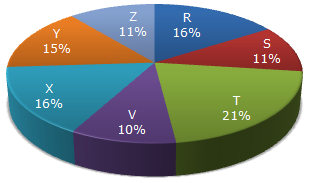# Data Interpretation - Pie Charts - Discussion

Study the following pie-chart and the table and answer the questions based on them.

Proportion of Population of Seven Villages in 1997Village % Population Below Poverty Line X 38 Y 52 Z 42 R 51 S 49 T 46 V 58

1.

If the population of village R in 1997 is 32000, then what will be the population of village Y below poverty line in that year?

 [A]. 14100 [B]. 15600 [C]. 16500 [D]. 17000

Explanation:

Population of village R = 32000 (given).

Let the population of village Y be y.

 Then, 16 : 15 = 32000 : yy =15 x 32000= 30000. 16Population of village Y below poverty line = 52% of 30000 = 15600.

 Suzuki said: (Jun 26, 2011) Great !

 Pooja Yadav said: (May 28, 2012) I want to know of hint for solving the pie chart questions.

 Vasavi said: (Feb 16, 2013) Here the given population is 32000 for Village R. From the pie chart it clearly mentioned the % of Village R is 16% of the total population, since we do not know the total population let we take it as "X". So now ; X + 16% = 32000. X= 32000*100/16 which is 2,Lkhs , So now the total Population was derived as 2,Lkh , For Village Y (Given %) => 15. So 2,00,000 * 15/100 = 30,000. From this 30,000 we have to find for the poverty Line % (given in the table) as 52%. So, 30,000*52/100 = 15600.

 Ilya said: (Apr 4, 2014) Why does it have to be village Y?

 Yara Ashraf said: (May 29, 2016) How to get 15% of population?

 Sk Kumar said: (Oct 19, 2016) How can you compare R and Y villages population?

 Shabbaz said: (Aug 10, 2017) It can be calculated as R=16% = 32000. ::Y=15%= So, 15% of 32000 = 30000, 52% is below poverty line for Y= 52% of 15%=7.8%, so, 30000*7.8/15 = 15600.

 Ruhi said: (Nov 9, 2018) How 16:15? Please explain.

 Sorav Kumar said: (Apr 25, 2019) Solve for S populatin given population of x is 12160 below the poverty line.